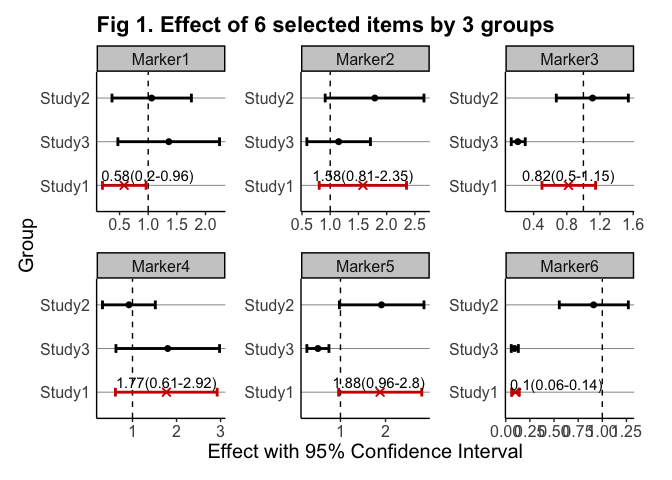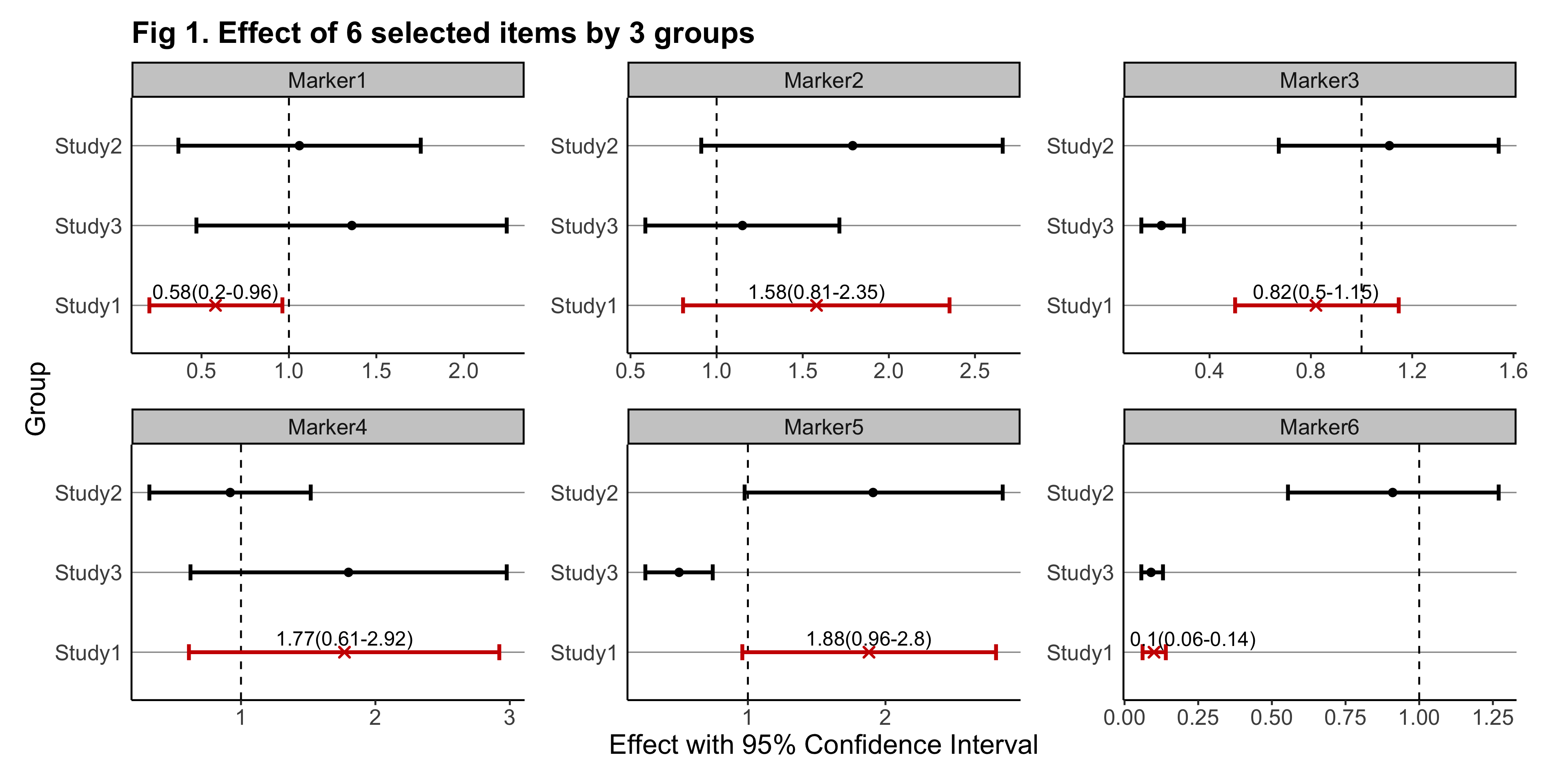# gwasforest

The goal of gwasforest is to extract and reform data from GWAS results, and then make a single integrated forest plot containing multiple windows of which each shows the result of individual SNPs (or other items of interest).

## Installation

The development version of gwasforest can be installed from GitHub with:

``devtools::install_github("yilixu/gwasforest", ref = "main")``

## Quick Demos

• ( 1 ) when customFilename (main input) is in dataframe format (with standardized column names):
``````library(gwasforest)
set.seed(123)

# generate example data
tempValue = runif(n = 18, min = 0.01, max = 2)
tempStdErr = tempValue / rep(3:5, times = 6)
eg_customFilename = data.frame(paste0("Marker", 1:6), tempValue[1:6], tempStdErr[1:6], tempValue[7:12], tempStdErr[7:12], tempValue[13:18], tempStdErr[13:18], stringsAsFactors = FALSE)
colnames(eg_customFilename) = c("MarkerName", paste0(rep("Study", times = 6), rep(1:3, each = 2), rep(c("__Value", "__StdErr"), times = 3)))
rm(tempValue, tempStdErr)``````
``````# run gwasforest function
eg_returnList = gwasforest(eg_customFilename, stdColnames = TRUE, valueFormat = "Effect", metaStudy = "Study1", colorMode = "duo")
#>  "Column names are in the same format as instruction example"
#>  "Loading user-provided values"
#>  "Start calculating Confidence Interval (non-exponential)"
#>  "Based on user's choice, GWAS results output file will not be generated"
#>  "All studies except meta study will be set in alphabetical order from top to bottom on the forest plot"
#> Registered S3 methods overwritten by 'ggplot2':
#>   method         from
#>   [.quosures     rlang
#>   c.quosures     rlang
#>   print.quosures rlang
#>  "Based on user's choice, GWAS forest plot file will not be generated"
#>  "Run completed, thank you for using gwasforest"``````
• ( 2 ) when customFilename is in dataframe format (without standardized column names), while customFilename_studyName is provided in dataframe format:
``````# generate example data
tempValue = runif(n = 18, min = 0.01, max = 2)
tempStdErr = tempValue / rep(3:5, times = 6)
eg_customFilename = data.frame(paste0("Marker", 1:6), tempValue[1:6], tempStdErr[1:6], tempValue[7:12], tempStdErr[7:12], tempValue[13:18], tempStdErr[13:18], stringsAsFactors = FALSE)
colnames(eg_customFilename) = c("MarkerName", paste0(rep("Study", times = 6), rep(1:3, each = 2), sample(LETTERS, 6)))
rm(tempValue, tempStdErr)
eg_customFilename_studyName = data.frame("studyName" = paste0("Study", 1:3), stringsAsFactors = FALSE)``````
``````# run gwasforest function
eg_returnList = gwasforest(eg_customFilename, customFilename_studyName = eg_customFilename_studyName, stdColnames = FALSE, customColnames = c("Value", "StdErr"), valueFormat = "Effect", metaStudy = "Study1", colorMode = "duo")``````
• ( 3 ) when customFilename_results is in dataframe format (run either of the two steps above to see the example results):
``````# extract results table
eg_customFilename_results = eg_returnList[]``````
``````library(ggplot2)

# render plot, see additional NOTES below
plot(eg_returnList[])
#> Warning: Removed 12 rows containing missing values (geom_text_repel).``````• ( 4 ) NOTES: As shown above, the plot rendered through plot() may suffer from certain issues such as low-resolution and overlapping labels. To overcome these issues and get the genuine plot output from gwasforest, it is recommended to provide a valid outputFolderPath so that a better-rendered plot can be created. The below is the genuine plot output created from the same example data: